Convex Pintegon Tilings

G4G Celebration of Mind     2021 October

Glen Whitney

studioinfinity.org/pintegons

Convex Pintegon Tilings

G4G Celebration of Mind     2021 October

Glen Whitney

studioinfinity.org/pintegons

Joint work with Alissa Crans

An old storyThe modern lookDeep rootsDeep rootsRegular tessellationsNo regular pentagons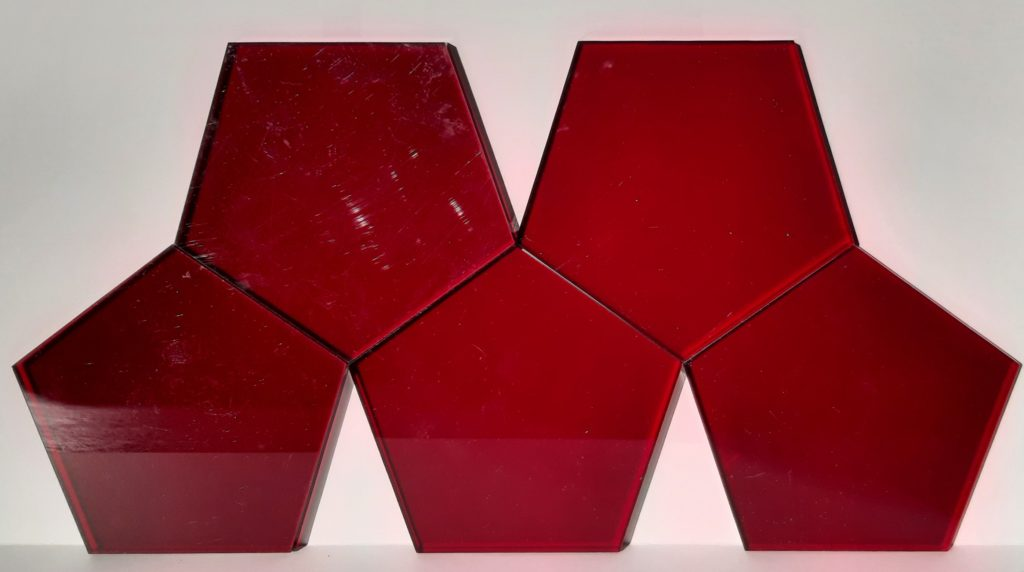No regular pentagons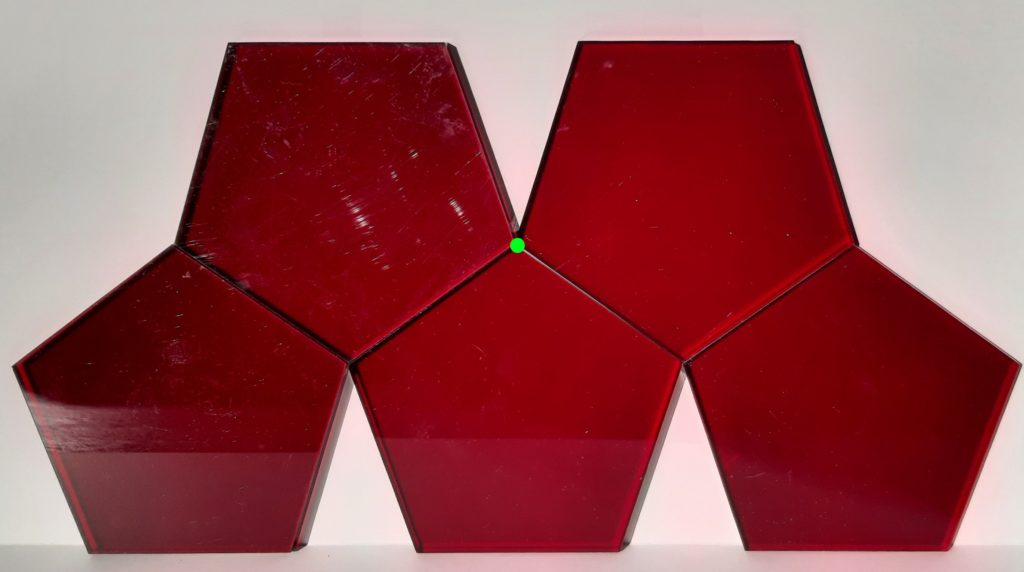No regular pentagons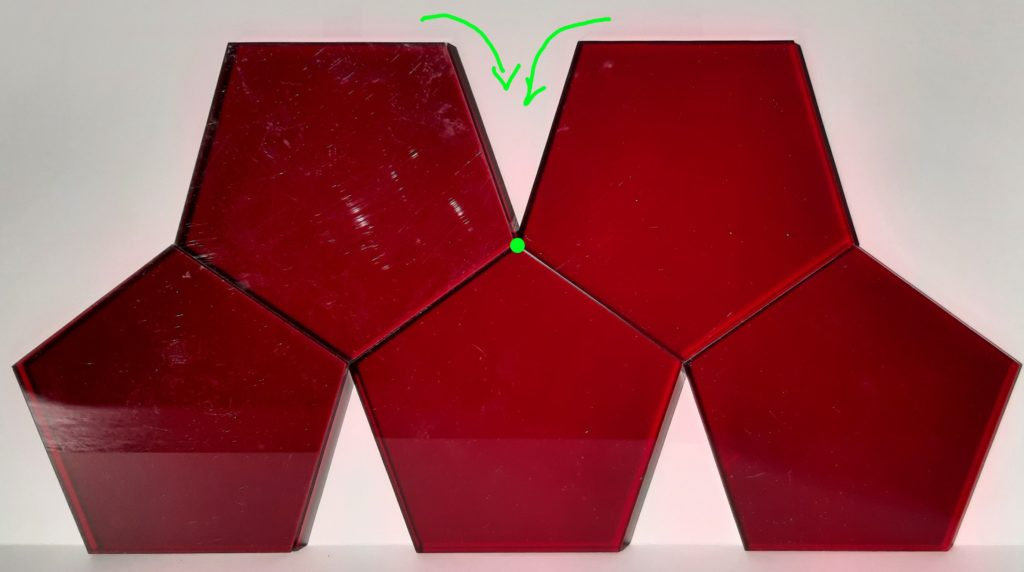Nevertheless…Mughal jali, India     c. 1615 c.e.

image from the Toledo Museum of Art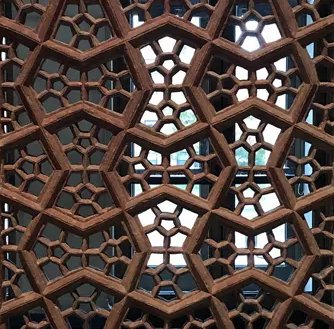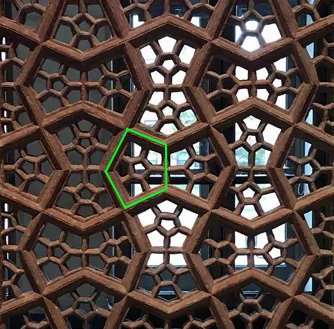Classic math move: Just relax

instead of insisting that our pentagons are regular, allow them to be merely convex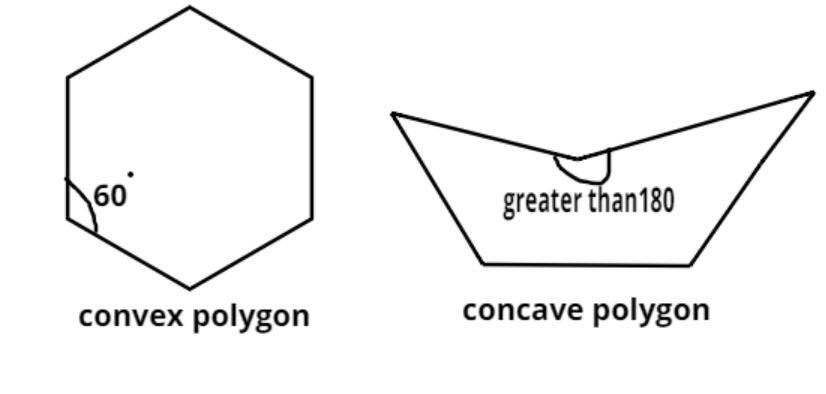What kinds of convex pentagons can tessellate?images from ‘kjo’ on math.stackexchange.com

A rich mathematical story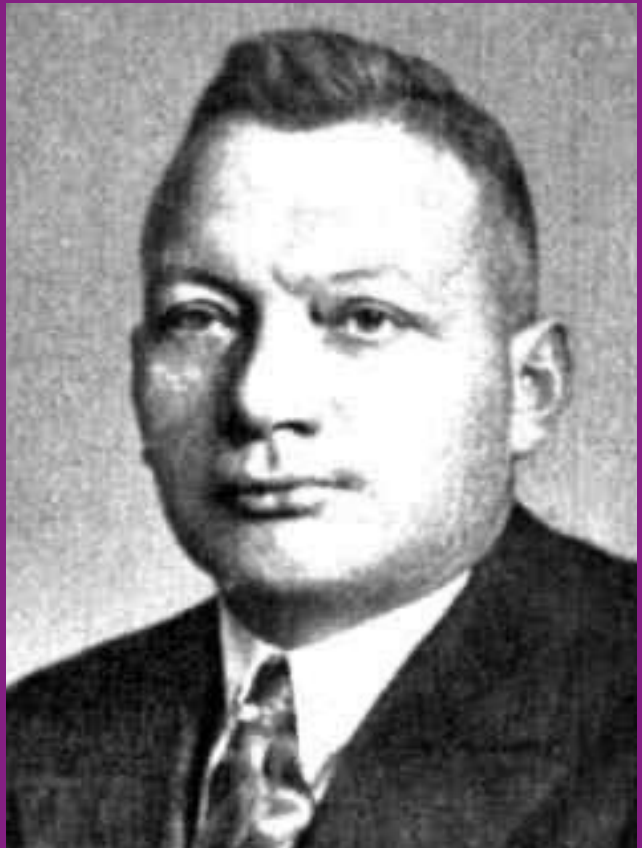1918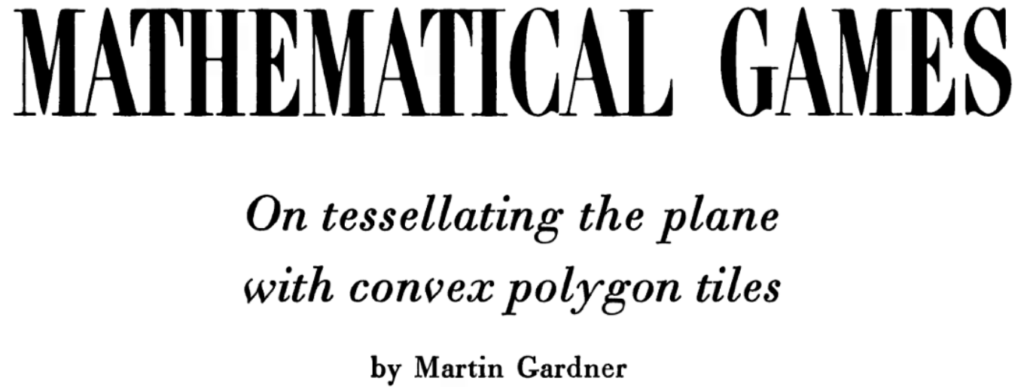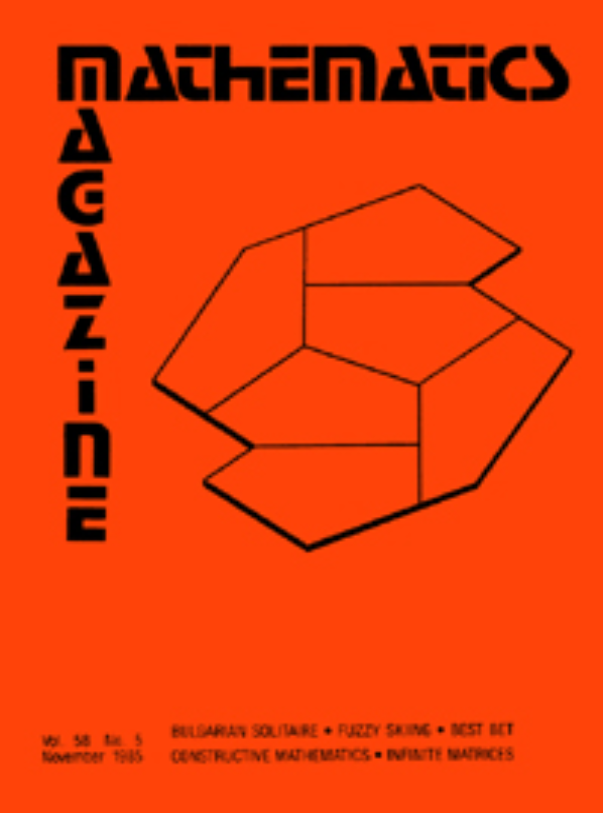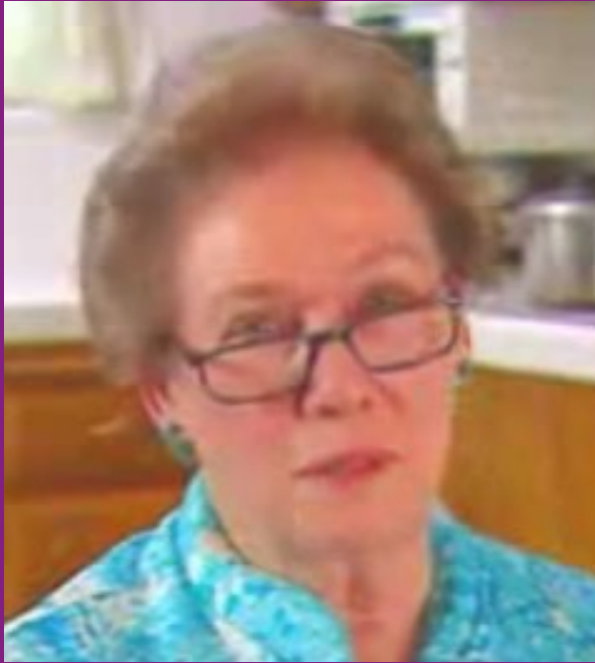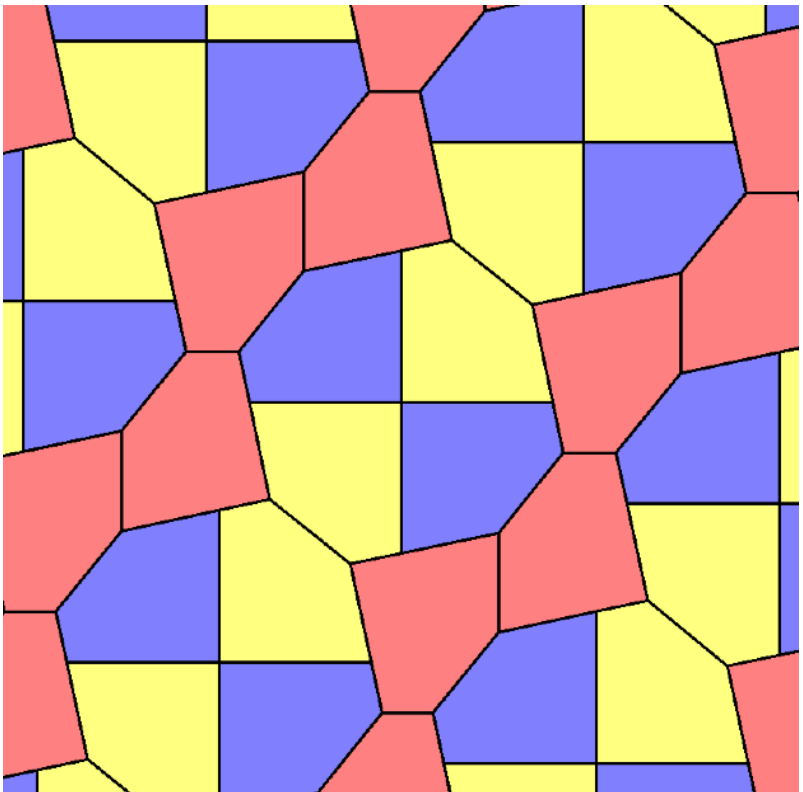Plus…  pretty pictures!Just certain kinds, called “families”, of convex pentagons tessellate

We’re looking for integer-sided pentagons in those familiesJust certain kinds, called “families”, of convex pentagons tessellate

We’re looking for pintegons in those familiesClassic math move: Constrain yourself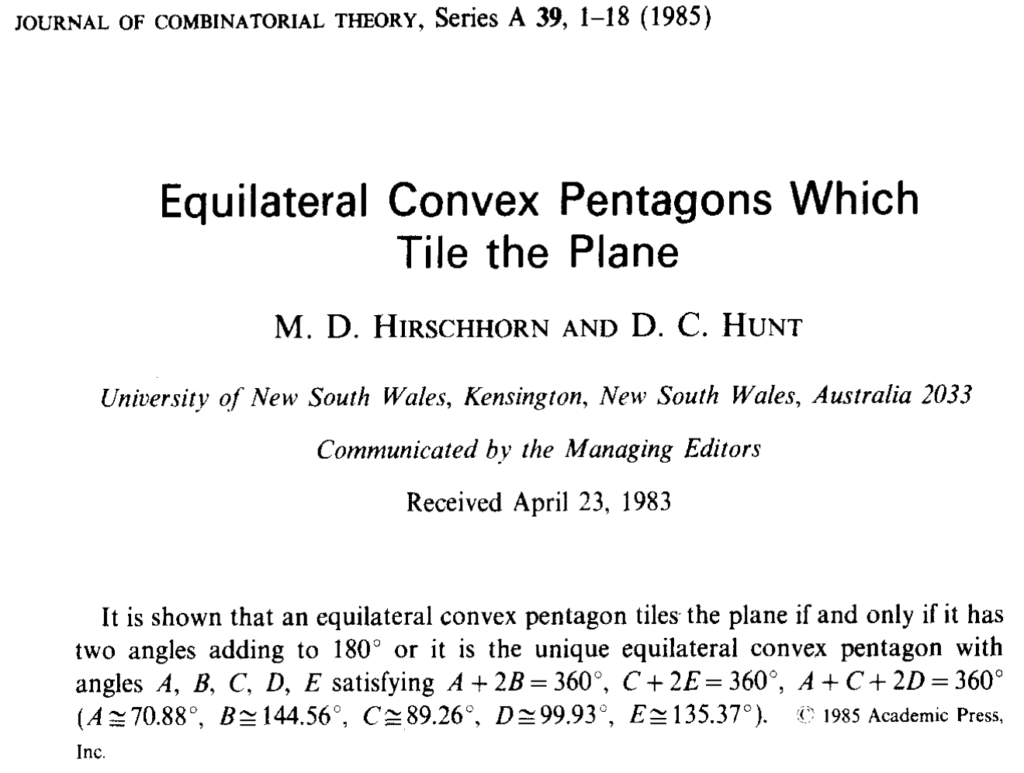Introducing Family 13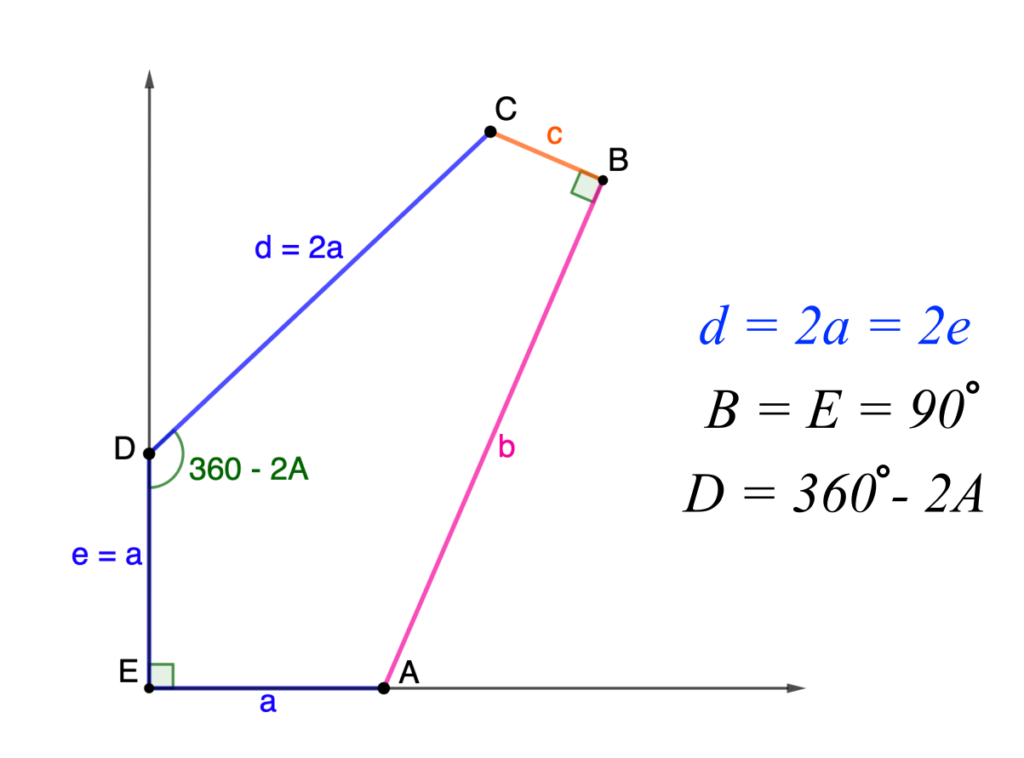Starting to look for pintegons…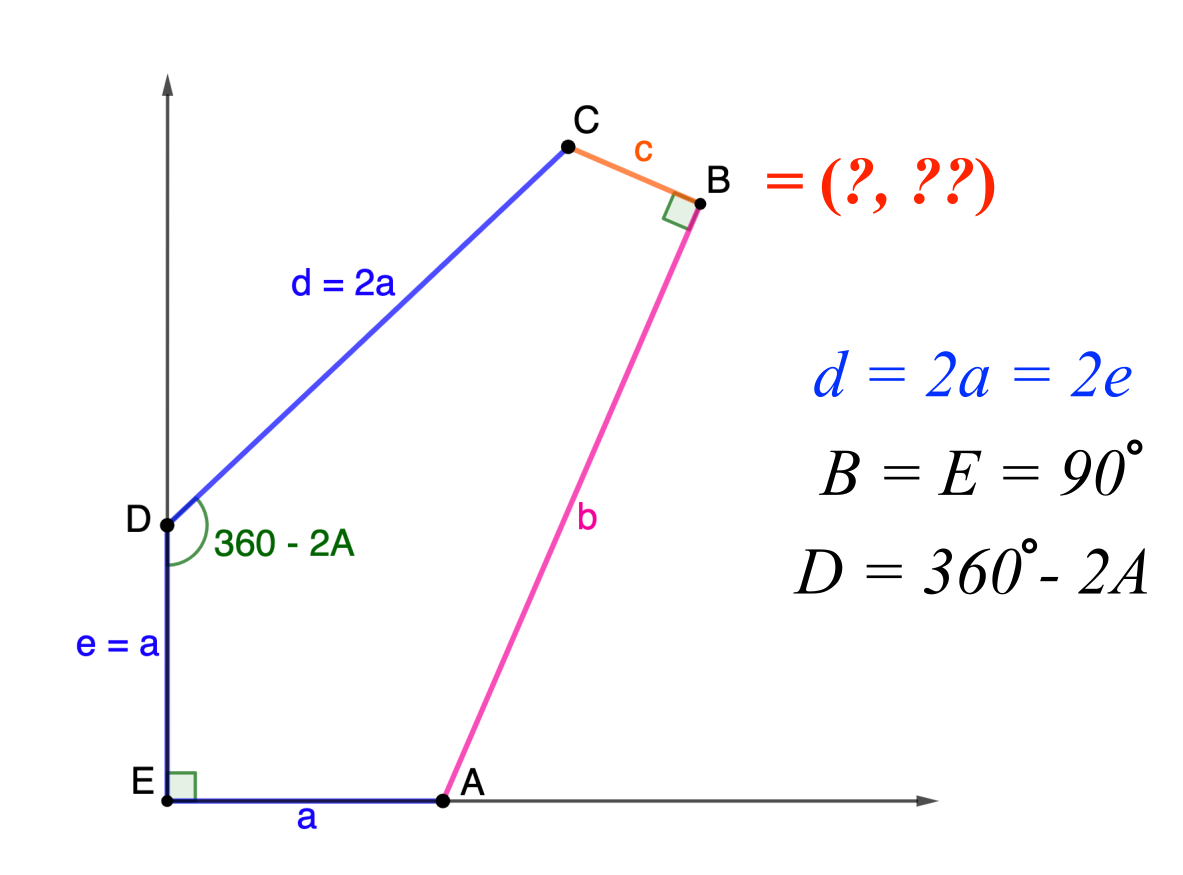Pintegons in Family 13Pintegons in Family 13Pintegons in Family 13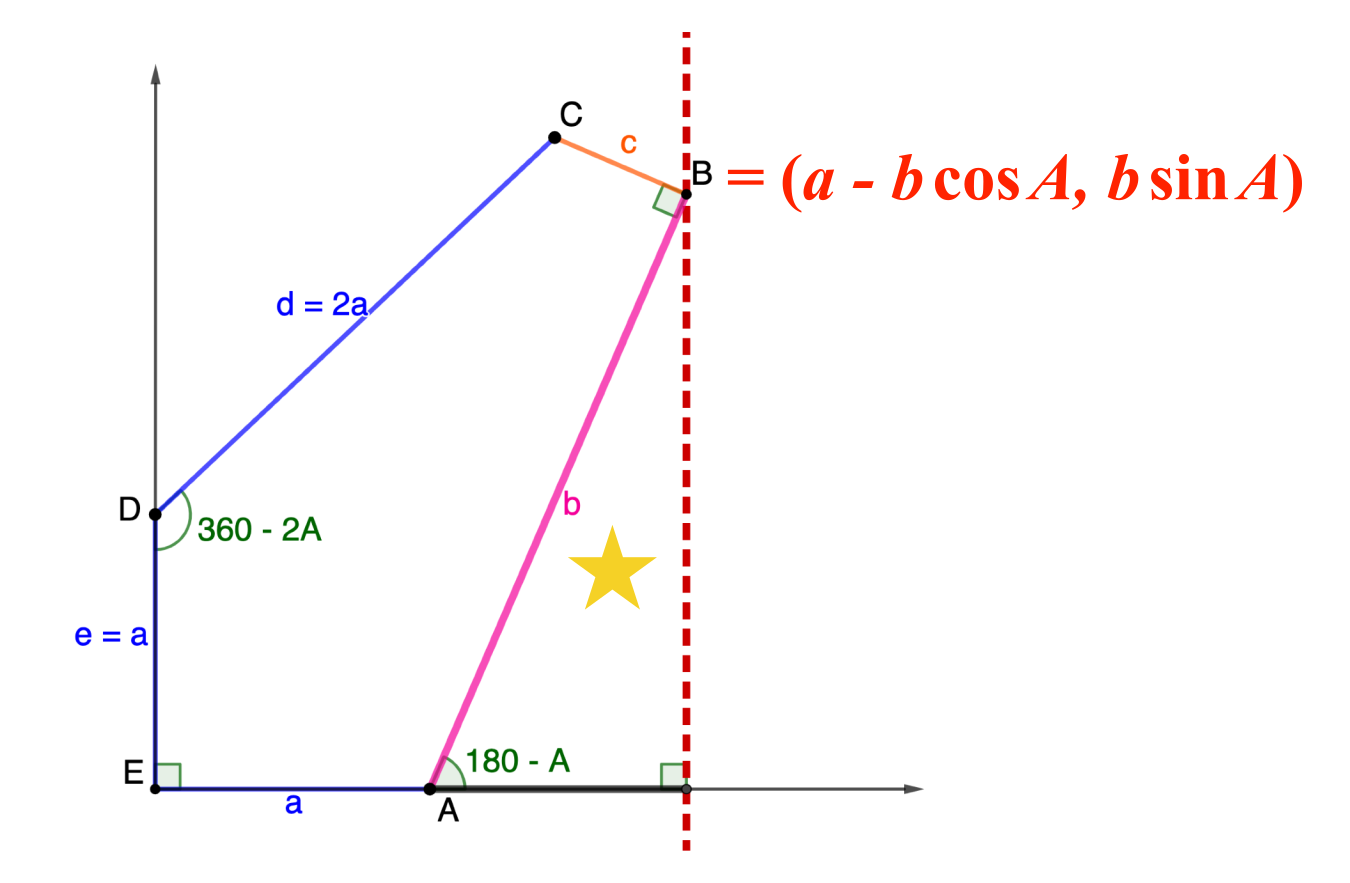Pintegons in Family 13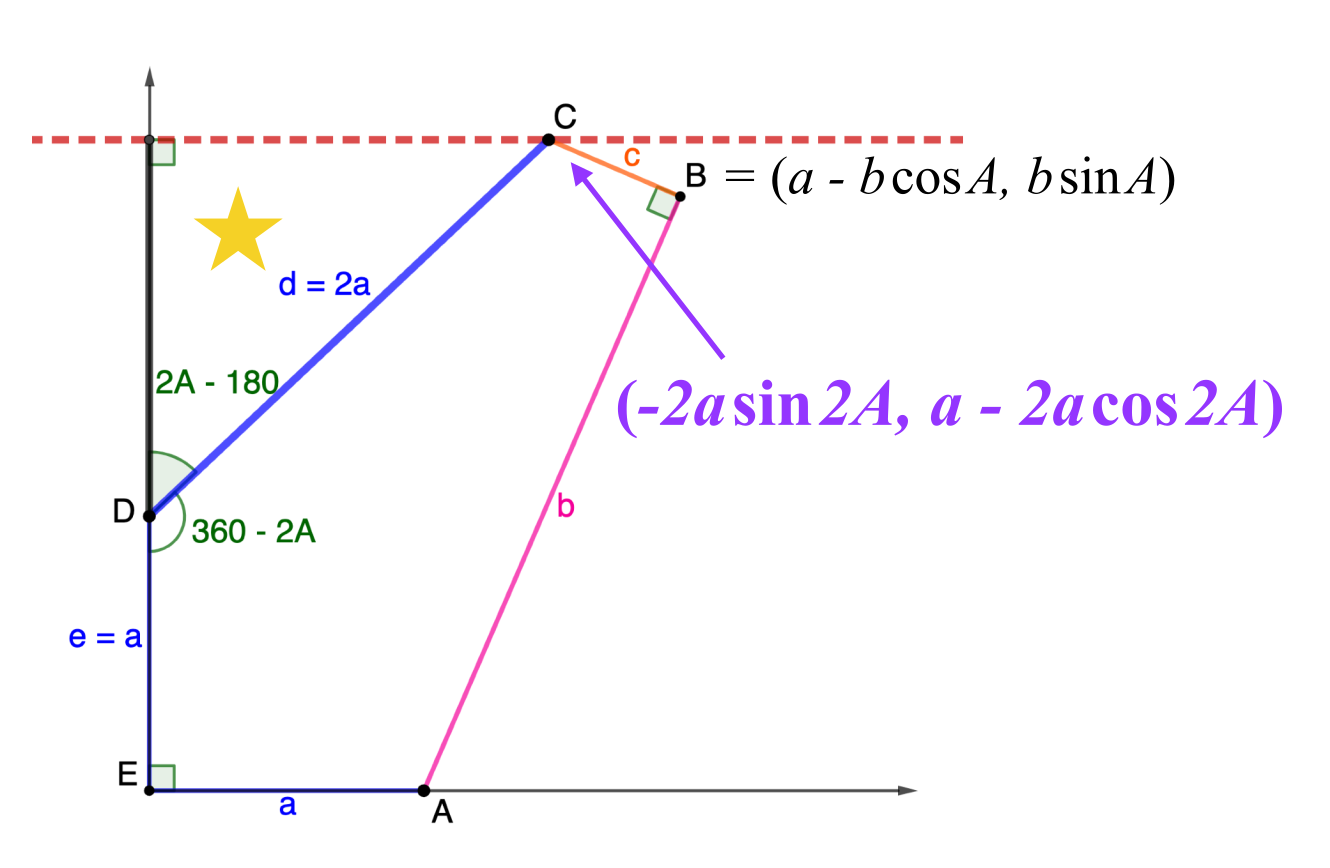Pintegons in Family 13Pintegons in Family 13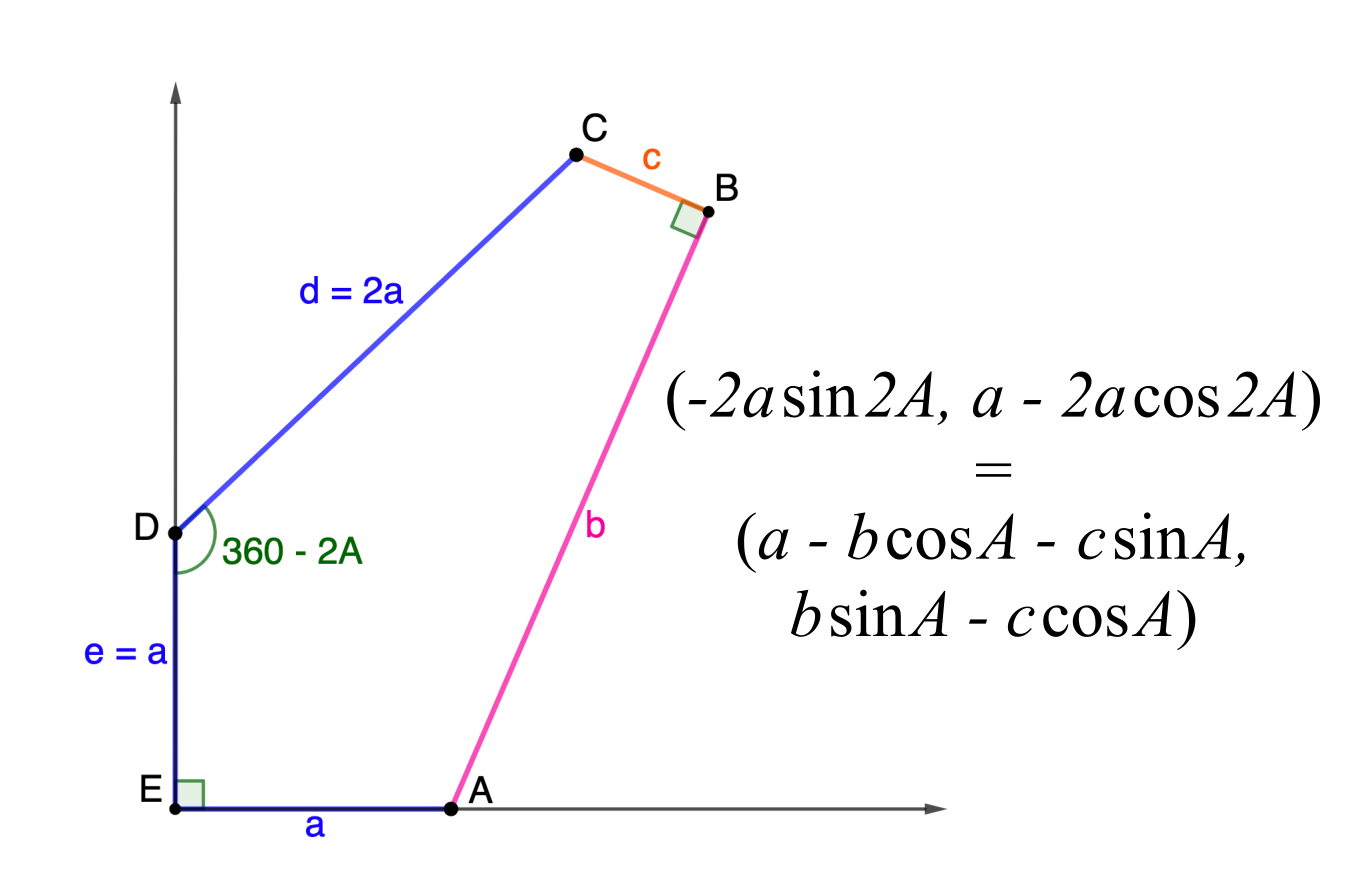Pintegons in Family 13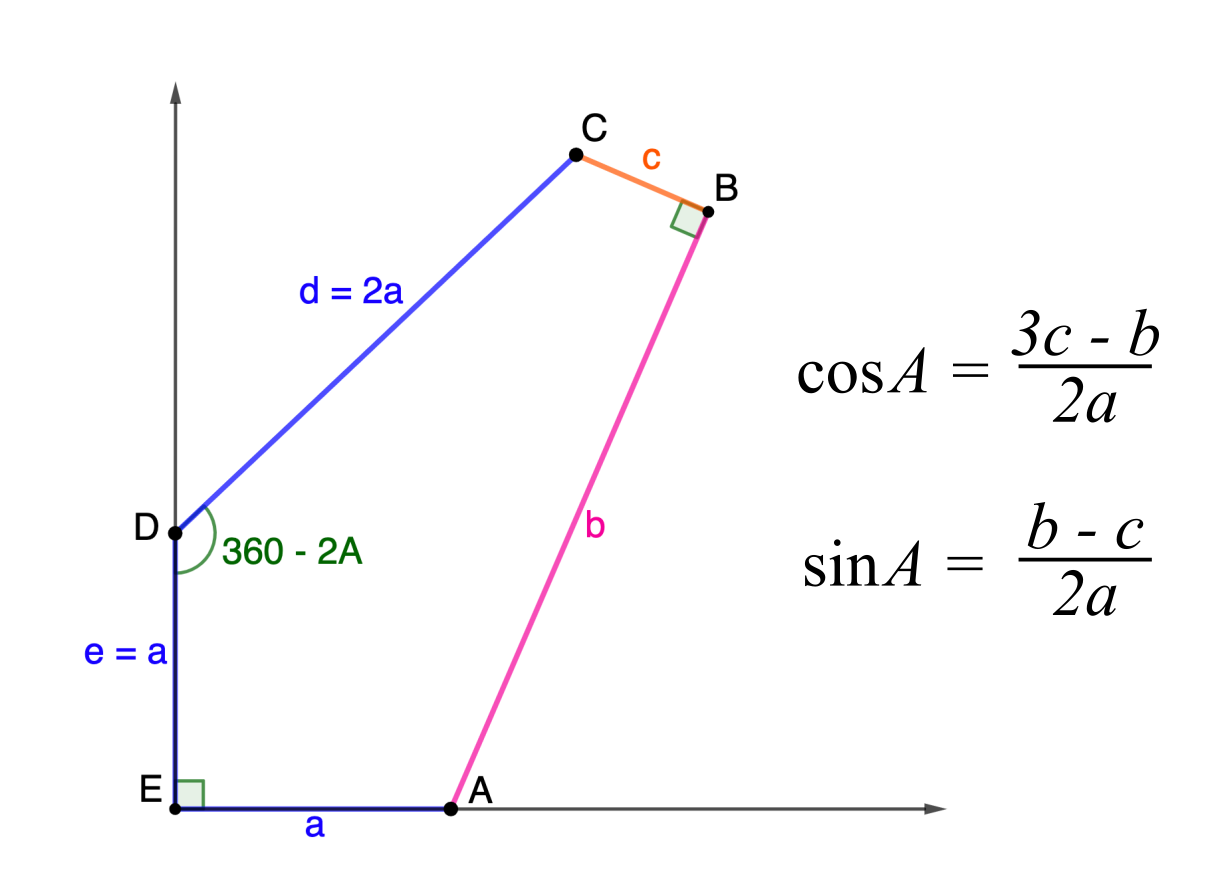Pintegons in Family 13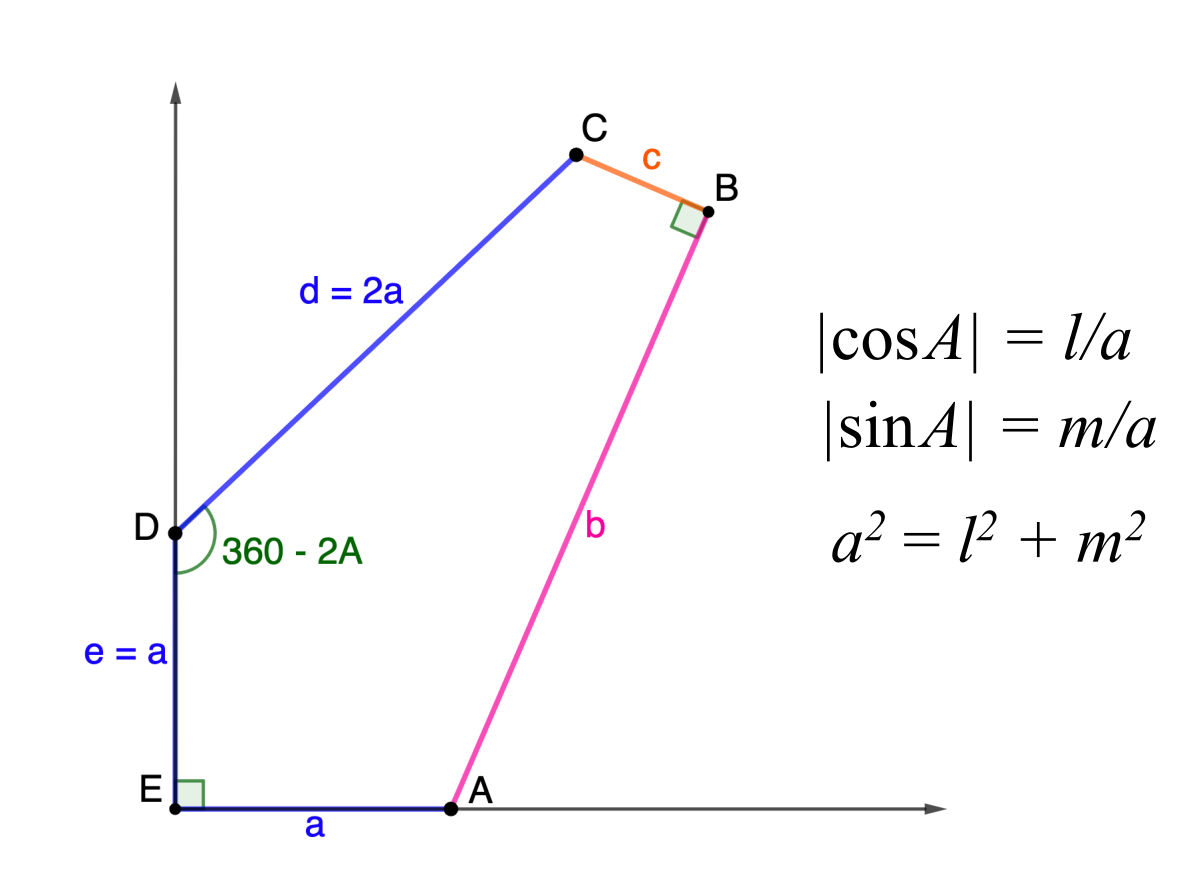Extreme “pintegons” in Family 13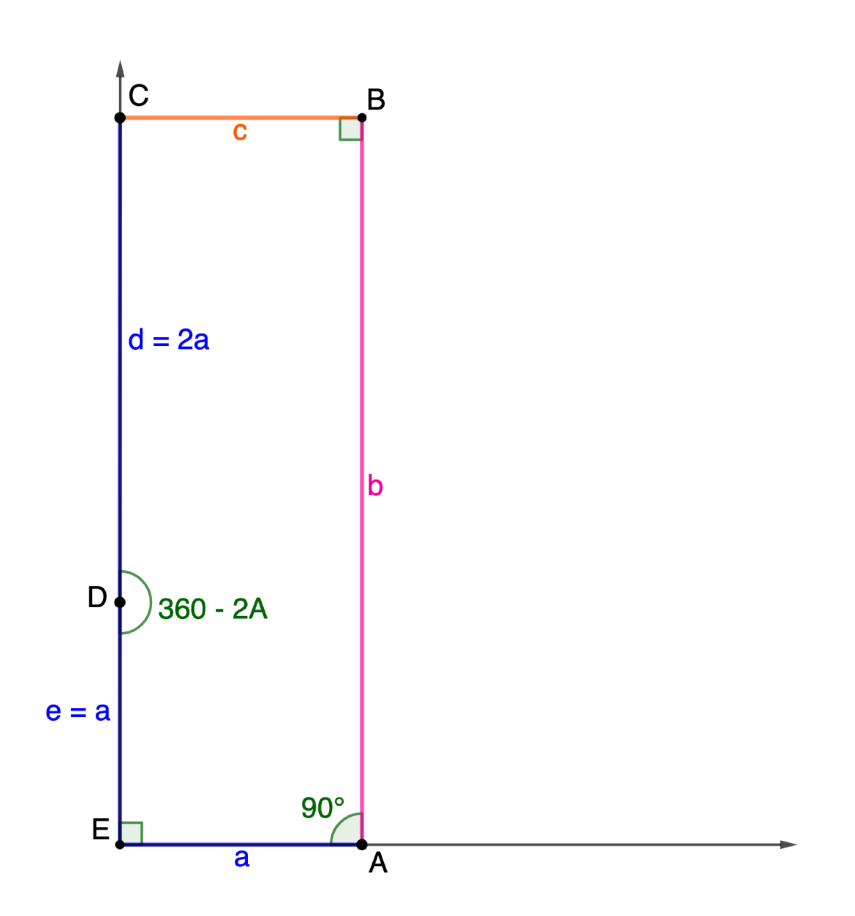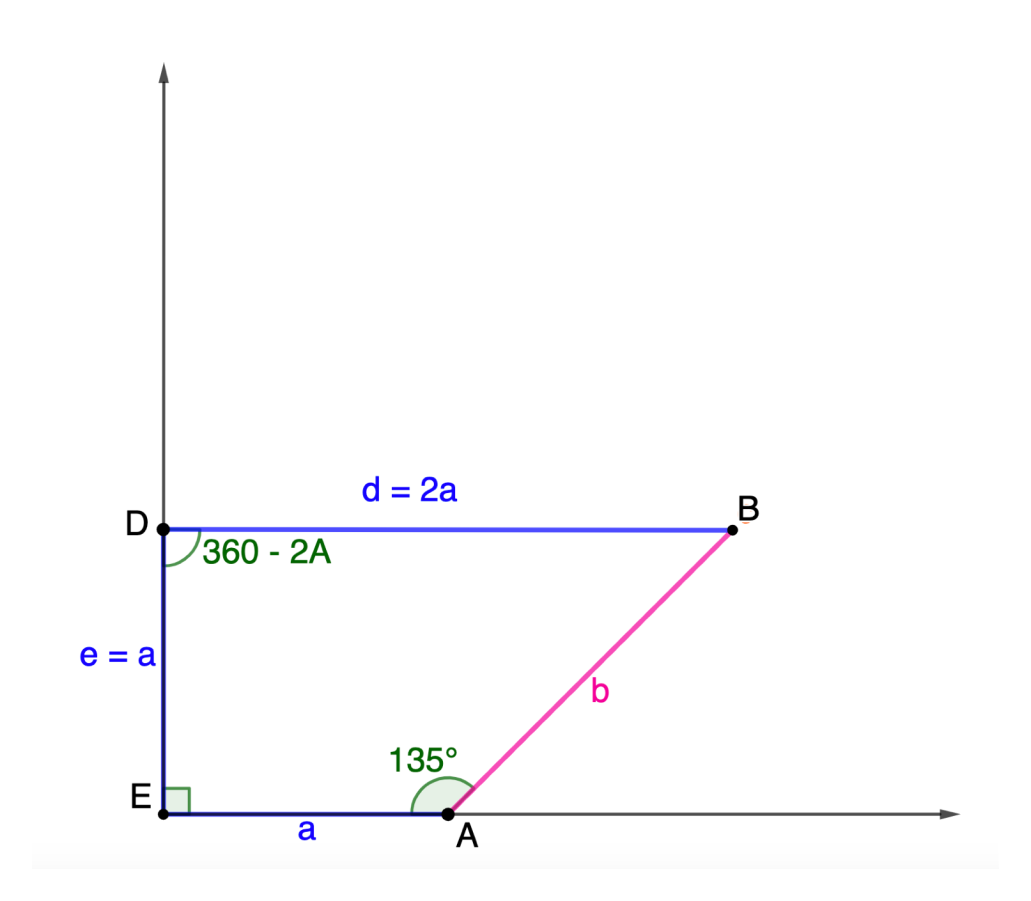Found the pintegons in Family 13!Not all families are alike…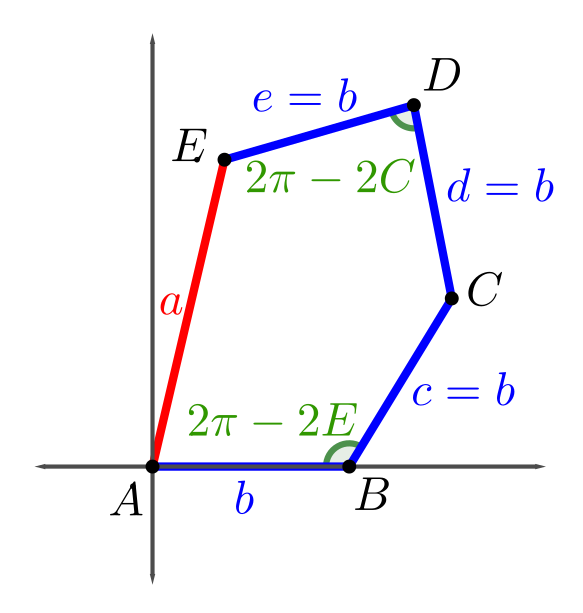Family 7
Upshot for Family 7The pintegons in Family 7 are those with sides $(a,b,b,b,b)$ for whole numbers $a$ and $b$ satisfying $0 < a < (2\sqrt\phi)b.$

Deeper waters…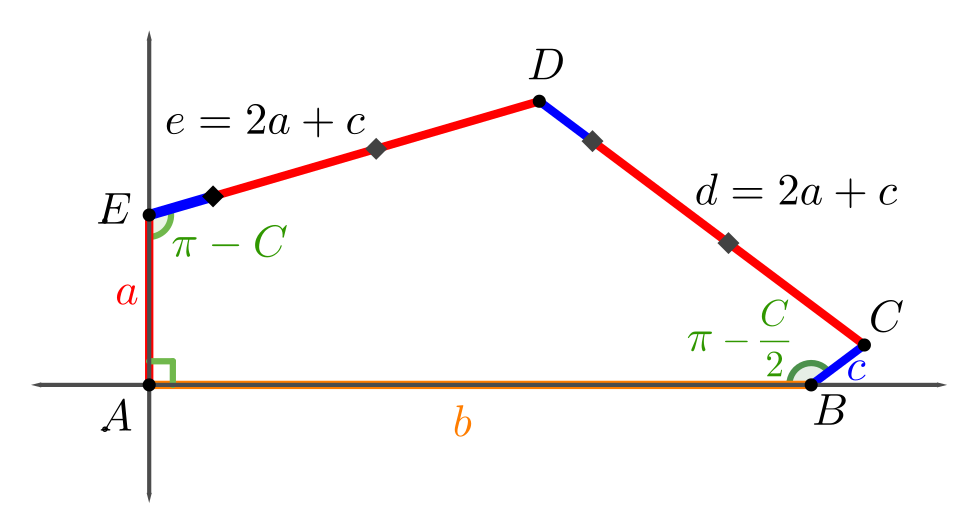Family 11
Analyzing Family 11$\begin{split}C: & \,\left(b+(d-2a)\cos\frac{C}2, (d-2a)\sin\frac{C}2\right) = & \,\left(d\left(\sin C + \cos\frac{C}2\right), a + d\left(\cos C − \sin\frac{C}2\right)\right) \end{split}$

Analyzing Family 11$\begin{split}b & = d \sin 2\gamma + 2a \cos \gamma 2(d − 2a) \sin \gamma & = a + d \cos 2\gamma − 2a \sin \gamma \end{split}$

Analyzing Family 11$\sin\gamma = \frac{a-d + \sqrt{a^2+3d^2}}{2d}$

Analyzing Family 11$H = a^2+3d^2$

$b = \frac{(\sqrt{H} + 3a − d)\sqrt{2(d − a)(\sqrt{H} + a)}}{2d}$

Detour into number theory

$H = a^2+3d^2$

When is $\sqrt{2(d − a)(\sqrt{H} + a)}$ of the form $r + s\sqrt{H}$ for rational numbers $r$ and $s$?

Detour into number theory

When is $\sqrt{2(d − a)(\sqrt{H} + a)}$ of the form $r + s\sqrt{H}$ for rational numbers $r$ and $s$?

Well, when is $\sqrt{n}$ of the form $r$ for a rational number $r$?

$\sqrt1, \sqrt2, \sqrt3, \sqrt4, \sqrt5, \sqrt6, \sqrt7, \sqrt8, \sqrt9, \sqrt{10}, \ldots$

Detour into number theory

When is $\sqrt{2(d − a)(\sqrt{H} + a)}$ of the form $r + s\sqrt{H}$ for rational numbers $r$ and $s$?

Answer: Only when $2(d − a)(\sqrt{H} + a) = (j + k\sqrt{H})^2$ for $j$ and $k$ each either a whole number or half of an odd number. (Dedekind, 1871)

Analyzing Family 11$H = a^2+3d^2$

Turns out $H$ must be a perfect square!

So there’s some $h$ such that $a^2 + 3d^2 = h^2.$

$b = \frac{(\sqrt{H} + 3a − d)\sqrt{2(d − a)(\sqrt{H} + a)}}{2d}$

Analyzing Family 11$H = a^2+3d^2$

Turns out $H$ must be a perfect square!

So there’s some $h$ such that $a^2 + 3d^2 = h^2.$

$b = \frac{(h + 3a − d)\sqrt{2(d − a)(h + a)}}{2d}$

Analyzing Family 11Integers $a, d, h,$ and $m$ so:

$a^2 + 3d^2 = h^2$

$2(d-a)(h+a) = m^2$

$h+3a-d > 0$

$b = \frac{(h+3a-d)\sqrt{2(d-a)(h + a)}}{2d}$

Family 11 pintegons are rare finds45
448
115
275
275
Family 11 pintegons are rare finds45
629
448
3750
115
1921
275
3179
275
3179
What remains?
More for you to explore…X
X

More for you to explore…X
X

studioinfinity.org/pintegons